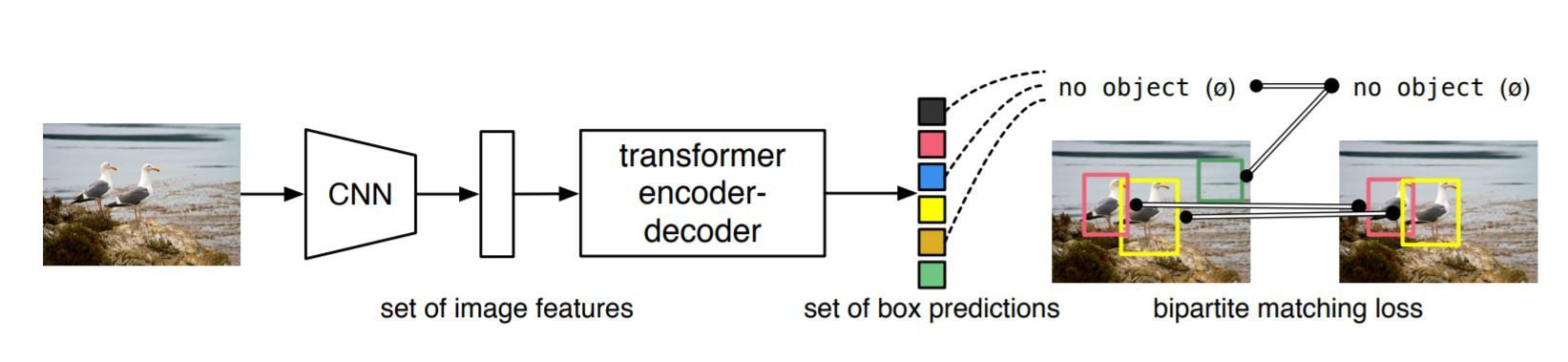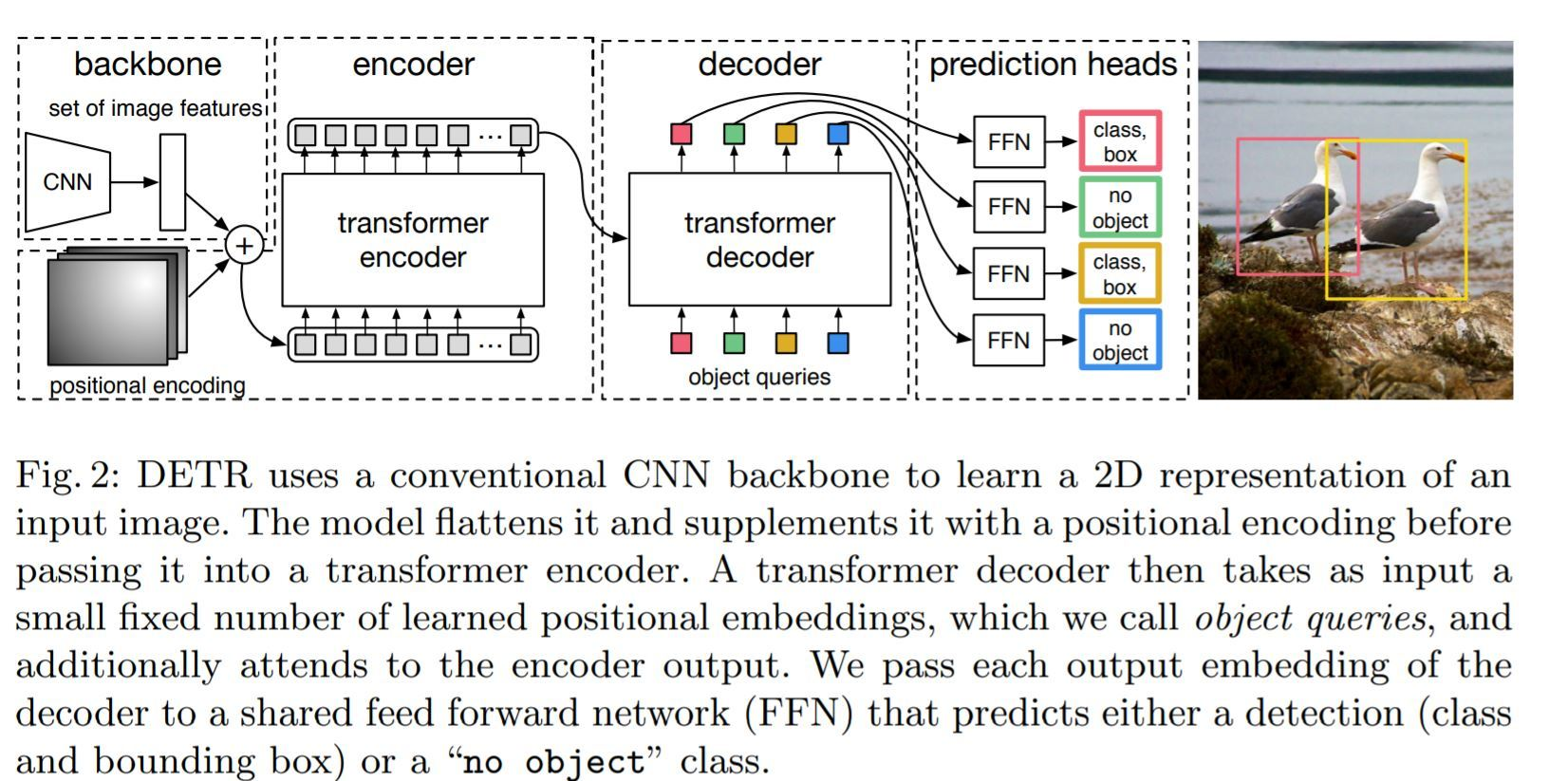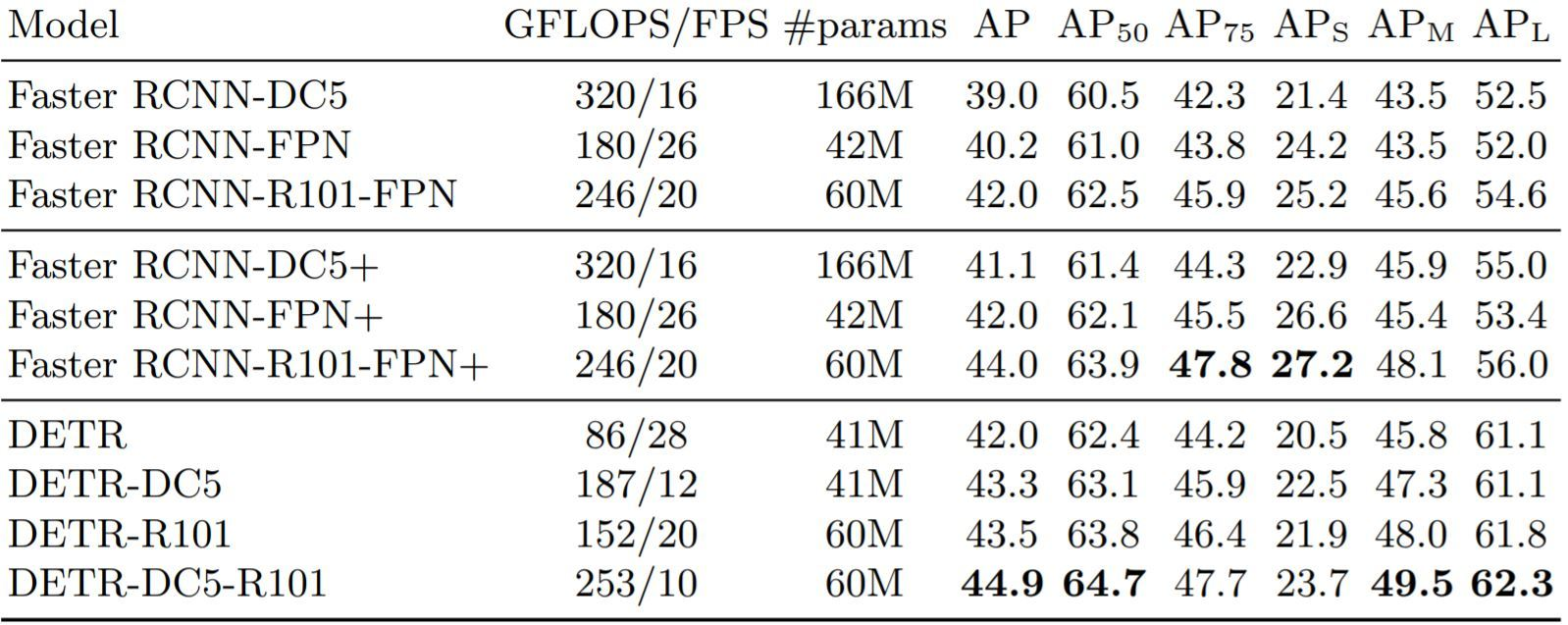# Object Detection with Detection Transformer (DERT) by Facebook

Facebook has just released its State of the art object detection Model on 27 May 2020. They are calling it DERT stands for Detection Transformer as it uses transformers to detect objects.This is the first time that transformer is used for such a task of Object detection along with a Convolutional Neural network. There are other Object detection models such as the RCNN family, YOLO(You Look Only Once) and SSD(Single Shot Detection) but none of them have ever used a transformer to achieve this task. The best part of this model is that due to the fact that it is using a transformer, it makes the architecture very simple unlike all the other techniques mentioned with has all kinds of hyperparameters and layers. So without further adieu, let’s get started.

What is object detection?
Given a photo if you need to determine if the photo has a single particular object you can do it by classification. but if you want to get the location of that object as well inside the image ….well even that is not an object detection task ….its called classification and localization. But if there are multiple objects in an image and you want the location of each and every location of every object, then that is object detection.
Some of the previous techniques try to get an RPN(Region Proposal Network) to come up with potential regions that may contain the object and then we can use the concept of anchor boxes, NMS(non-max-suppression)and IOU to generate relevant boxes and identify the object. Although these concepts work it takes some time for inferencing so real-time use with high accuracy is not achieved due to its complexity.
On a high level, this uses CNN and then a transformer to detect an object and it does so via a bipartite matching training object. This is the main reason why it is so simple.Source – https://arxiv.org/pdf/2005.12872.pdf

Step 1:
We put the image through a convolution Neural Network Encoder because CNN works best with images. So after passing through CNN the image features are conserved. This is the higher-order representation of an image with many more feature channels.
Step 2:
This enriched feature map of the image is given to a transformer encoder-decoder, which outputs the set of box prediction. Each of these boxes is consisting of a tuple. The tuple will be a class and a bounding box. Note: this also includes the class NULL or Nothing class and its position as well.

Now, this is a real problem as in the annotation there is no object class annotated as nothing. Comparing and dealing with similar objects next to each other is another major issue and in this paper, it is tackled by using bipartite matching loss. The loss is compared by comparing each class and bounding box there is with its corresponding class and box including the none class, which are let’s say N, with the annotation including the part added that contains nothing to make the total boxes N. The assignment of the predicted to the actual is a one to one assignment such that the total loss is minimized. There is a very famous algorithm called the Hungarian method to compute these minimum matching.
The main components:source – https://arxiv.org/pdf/2005.12872.pdf

The backbone – Features extracted from a Convolutional Neural Network and a positional encoding are passed
The transformer Encoder – A transformer is naturally a sequence processing unit and for the same reason, we the incoming tensors are flattened. It transforms the sequence into an equally long sequence of features.

The Transformer Decoder – takes in Object queries So its a decoder as a side input for conditioning information.

Prediction Feed-Forward Network (FFN) – The output for this is going through a classifier which outputs the class labels and bounding box output discussed earlier

Evaluator:
The evaluation is done on COCO dataset and its primary competitor was the RCNN family that has ruled this category for quiet some time and is considered to be the most classic technique for object detection.Source – https://arxiv.org/pdf/2005.12872.pdf

Pros:

• This new model is quite simple and you don’t have to install any library to use it.
• DETR demonstrates significantly better performance on large objects and not on a small object which can be further improved.
• A good thing is that they have even provided the code in the paper so now we will also implement it to know what its really capable of doing.

Code:

 `# Write Python3 code here ` `mport torch ` `from` `torch ``import` `nn ` `from` `torchvision.models ``import` `resnet50 ` ` `  `class` `DETR(nn.Module): ` ` `  `def` `__init__(``self``, num_classes, hidden_dim, nheads, ` `num_encoder_layers, num_decoder_layers): ` `  ``super``().__init__() ` `  ``# We take only convolutional layers from ResNet-50 model ` `  ``self``.backbone ``=` `nn.Sequential(``*``list``(resnet50(pretrained``=``True``).children())[:``-``2``]) ` `  ``self``.conv ``=` `nn.Conv2d(``2048``, hidden_dim, ``1``) ` `  ``self``.transformer ``=` `nn.Transformer(hidden_dim, heads, ` `  ``num_encoder_layers, num_decoder_layers) ` `  ``self``.linear_class ``=` `nn.Linear(hidden_dim, num_classes ``+` `1``) ` `  ``self``.linear_bbox ``=` `nn.Linear(hidden_dim, ``4``) ` `  ``self``.query_pos ``=` `nn.Parameter(torch.rand(``100``, hidden_dim)) ` `  ``self``.row_embed ``=` `nn.Parameter(torch.rand(``50``, hidden_dim ``/``/` `2``)) ` `  ``self``.col_embed ``=` `nn.Parameter(torch.rand(``50``, hidden_dim ``/``/` `2``)) ` `  ``def` `forward(``self``, inputs): ` `  ``x ``=` `self``.backbone(inputs) ` `  ``h ``=` `self``.conv(x) ` `  ``H , W ``=` `h.shape[``-``2``:] ` `  ``pos ``=` `torch.cat([ ` `  ``self``.col_embed[:W].unsqueeze(``0``).repeat(H, ``1``, ``1``), ` `  ``self``.row_embed[:H].unsqueeze(``1``).repeat(``1``, W, ``1``), ` `], dim``=``-``1``).flatten(``0``, ``1``).unsqueeze(``1``) ` `  ``h ``=` `self``.transformer(pos ``+` `h.flatten(``2``).permute(``2``, ``0``, ``1``), ` `  ``self``.query_pos.unsqueeze(``1``)) ` `  ``return` `self``.linear_class(h), ``self``.linear_bbox(h).sigmoid() ` `detr ``=` `DETR(num_classes``=``91``, hidden_dim``=``256``, nheads``=``8``, num_encoder_layers``=``6``, num_decoder_layers``=``6``) ` `detr.``eval``() ` `inputs ``=` `torch.randn(``1``, ``3``, ``800``, ``1200``) ` `logits, bboxes ``=` `detr(inputs) ` ` `  ` `  `Listing ``1``: <``/``strong>DETR PyTorch inference code. For clarity, it uses learned positional encodings ``in` `the encoder instead of fixed, ``and` `positional encodings are added to the ``input` `only instead of at each transformer layer. Making these changes requires going beyond ` `PyTorch implementation of transformers, which hampers readability. The entire code ` `to reproduce the experiments will be made available before the conference. `

We take only convolutional layers from ResNet-50 model
Code taken from the paper

Code: Try running this code on colab or just go to this link, copy and run the complete file.

 `import` `torch as th ` `import` `torchvision.transforms as T ` `import` `requests ` `from` `PIL ``import` `Image, ImageDraw, ImageFont `

We will be using ResNet 101 as the backbone architecture and we will be loading this architecture directly from the Pytorch Hub.
Code:

 `model ``=` `th.hub.load(``'facebookresearch/detr'``, ``'detr_resnet101'``, pretrained``=``True``) ` `model.``eval``() ` `model ``=` `model.cuda() `

 `# standard PyTorch mean-std input image normalization ` `transform ``=` `T.Compose([ ` `    ``T.ToTensor(), ` `    ``T.Normalize([``0.485``, ``0.456``, ``0.406``], [``0.229``, ``0.224``, ``0.225``]) ` `]) ` ` `  `CLASSES ``=` `[ ` `    ``'N/A'``, ``'person'``, ``'bicycle'``, ``'car'``, ``'motorcycle'``, ``'airplane'``, ``'bus'``, ` `    ``'train'``, ``'truck'``, ``'boat'``, ``'traffic light'``, ``'fire hydrant'``, ``'N/A'``, ` `    ``'stop sign'``, ``'parking meter'``, ``'bench'``, ``'bird'``, ``'cat'``, ``'dog'``, ``'horse'``, ` `    ``'sheep'``, ``'cow'``, ``'elephant'``, ``'bear'``, ``'zebra'``, ``'giraffe'``, ``'N/A'``, ``'backpack'``, ` `    ``'umbrella'``, ``'N/A'``, ``'N/A'``, ``'handbag'``, ``'tie'``, ``'suitcase'``, ``'frisbee'``, ``'skis'``, ` `    ``'snowboard'``, ``'sports ball'``, ``'kite'``, ``'baseball bat'``, ``'baseball glove'``, ` `    ``'skateboard'``, ``'surfboard'``, ``'tennis racket'``, ``'bottle'``, ``'N/A'``, ``'wine glass'``, ` `    ``'cup'``, ``'fork'``, ``'knife'``, ``'spoon'``, ``'bowl'``, ``'banana'``, ``'apple'``, ``'sandwich'``, ` `    ``'orange'``, ``'broccoli'``, ``'carrot'``, ``'hot dog'``, ``'pizza'``, ``'donut'``, ``'cake'``, ` `    ``'chair'``, ``'couch'``, ``'potted plant'``, ``'bed'``, ``'N/A'``, ``'dining table'``, ``'N/A'``, ` `    ``'N/A'``, ``'toilet'``, ``'N/A'``, ``'tv'``, ``'laptop'``, ``'mouse'``, ``'remote'``, ``'keyboard'``, ` `    ``'cell phone'``, ``'microwave'``, ``'oven'``, ``'toaster'``, ``'sink'``, ``'refrigerator'``, ``'N/A'``, ` `    ``'book'``, ``'clock'``, ``'vase'``, ``'scissors'``, ``'teddy bear'``, ``'hair drier'``, ` `    ``'toothbrush'` `] `

Enter the URL of an image here. The one I have used is https://i.ytimg.com/vi/vrlX3cwr3ww/maxresdefault.jpg
Code:

 `url ``=` `input``() `

Displaying the image

 `img ``=` `Image.``open``(requests.get(url, stream``=``True``).raw).resize((``800``,``600``)).convert(``'RGB'``) ` `img `

Code:

 `img_tens ``=` `transform(img).unsqueeze(``0``).cuda() ` `with th.no_grad(): ` `  ``output ``=` `model(img_tens) ` ` `  `draw ``=` `ImageDraw.Draw(img) ` `pred_logits``=``output[``'pred_logits'``][``0``][:, :``len``(CLASSES)] ` `pred_boxes``=``output[``'pred_boxes'``][``0``] ` ` `  `max_output ``=` `pred_logits.softmax(``-``1``).``max``(``-``1``) ` `topk ``=` `max_output.values.topk(``15``) ` ` `  `pred_logits ``=` `pred_logits[topk.indices] ` `pred_boxes ``=` `pred_boxes[topk.indices] ` `pred_logits.shape `

Code:

 `for` `logits, box ``in` `zip``(pred_logits, pred_boxes): ` `  ``cls` `=` `logits.argmax() ` `  ``if` `cls` `>``=` `len``(CLASSES): ` `    ``continue` `  ``label ``=` `CLASSES[``cls``] ` `  ``print``(label) ` `  ``box ``=` `box.cpu() ``*` `th.Tensor([``800``, ``600``, ``800``, ``600``]) ` `  ``x, y, w, h ``=` `box ` `  ``x0, x1 ``=` `x``-``w``/``/``2``, x``+``w``/``/``2` `  ``y0, y1 ``=` `y``-``h``/``/``2``, y``+``h``/``/``2` `  ``draw.rectangle([x0, y0, x1, y1], outline``=``'red'``, width``=``5``) ` `  ``draw.text((x, y), label, fill``=``'white'``) `

Code: Displaying the detected image

 `img `

Here is the link to the colab notebook and github code. Also, feel free to check out the official GitHub for the same
Drawbacks:
It takes forever to train. It trained for six days on 8 GPUs. It’s not that much when you compare it with the language model at this scale as they use a transformer but still.

My Personal Notes arrow_drop_upCheck out this Author's contributed articles.

If you like GeeksforGeeks and would like to contribute, you can also write an article using contribute.geeksforgeeks.org or mail your article to contribute@geeksforgeeks.org. See your article appearing on the GeeksforGeeks main page and help other Geeks.

Please Improve this article if you find anything incorrect by clicking on the "Improve Article" button below.